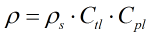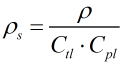# C023 – API Density Referral 1980-86

## Description

This converts density values between standard conditions (1.01325 bar and 15°C) and other observed or line conditions.

The correction for temperature is calculated using the Manual of Petroleum Measurement Standards Chapter 11.1 – Volume correction factors Volume 10 – Background, Development and Program Documentation: API Standard 2540 First Edition August 1980.

Measurement of Petroleum Measurement Standards Chapter 11.2.1 – Compressibility Factors for Hydrocarbons: 0-90° API Gravity Range (1984)

Measurement of Petroleum Measurement Standards Chapter 11.2.1M – Compressibility Factors for Hydrocarbons: 638-1074 Kilograms per Cubic Metre Range (First Edition August 1984)

Measurement of Petroleum Measurement Standards Chapter 11.2.2 – Compressibility Factors for Hydrocarbons: 0.350-0.637 Relative Density (60°F/60°F) and -50°F to 140°F Metering Temperature (First Edition October 1986)

Measurement of Petroleum Measurement Standards Chapter 11.2.2M – Compressibility Factors for Hydrocarbons: 350-637 Kilograms per Cubic Metre Density (15°C) and -46°C to 60°C Metering Temperature (First Edition October 1986)

Kelton calculation reference C023

FLOCALC calculation reference F023

KIMS calculation reference K212

## Options

### Solve for

The standard density calculation methods (with the exception of User entered RD) are bi directional

• Standard density

Calculate the standard density from the density at a given pressure and temperature

• Density

Calculate the density at a given pressure and temperature from the standard density

### Precision

The precision options enables switching between rounding methods

• Full

This enables you to bypass the rounding methods outlined in the algorithms and use the full precision during the calculation

• Rounding

Select this option to follow the algorithms to the same rounding precision as is in the standard

## Calculation

The temperature correction factor, Ctl, is calculated using the procedure in C222.

The pressure correction factor, Cpl, is calculated using the procedure in C220.

### Density

When solving for density the temperature correction according to API 11.1 is applied followed by the pressure correction according to API MPMS Chapter 11.2.1.### Standard Density

When solving for standard density an iterative calculation is performed making use of the API 11.1 and API MPMS Chapter 11.2.1 algorithms. An initial estimate is taken for standard density based upon the entered density. This value is then fed into the API 11.1 temperature correction algorithm to gain the Ctl. The estimate for standard density is then taken into the API 11.2.1 pressure correction algorithm to gain the Cpl. The Ctl and Cpl are then fed into the below formula to gain a new estimate of standard density. The process is repeated until standard density values converge to within 10-6.Back